# Coding projectiles for your tower defense game — Part 2

In the second part of this series, we will be exploring how to give our projectiles a nice vertical arc as they travel towards the target. This article will expand upon the work in Part 1, where we coded a projectile that could home in onto the target and detect collision with the target without using Unity’s physics engine’s.

## What are projectile arcs?

These. Notice how the projectile arcs down onto its target?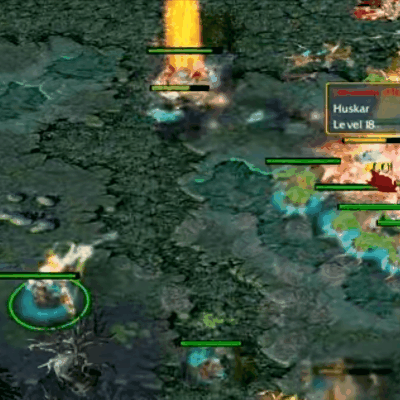Bone Clinkz from Warcraft III DotA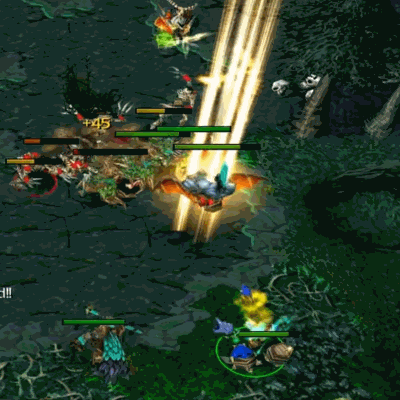Goblin Techies from Warcraft III DotA

To control the projectile’s height over the distance it travels, we are going to have to create a mathematical equation that graphs out in the shape of a curve.

## A brief primer on graphs

For the uninitiated, graphs are made by creating an equation that relates 2 variables (normally called x and y) together. They describe how a change in one variable affects another.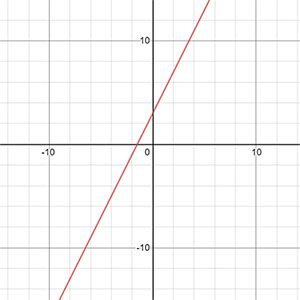y = 2x + 3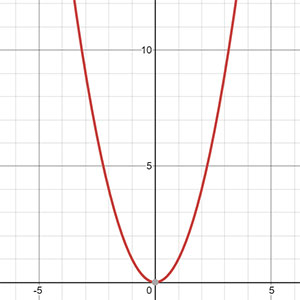y = x2

Take the y = 2x + 3 graph for instance: it is saying that the value of y is always equals to double of x, plus 3. For example, when x = 3:

We substitute x with 3 to get…

y = 2 × 3 + 3
y = 6 + 3 = 9

Another example, x = 6:

y = 2 × 6 + 3
y = 12 + 3 = 15

The point here is, for any value of x, there will be a corresponding value of y, and vice versa. By charting this relation out on a graph, we can capture the rate of change of y in relation to x.

Article continues after the advertisement:

## What does it matter to us?

Notice that to create a projectile arc, we will have to capture the relation between 1) the distance travelled by the projectile; and 2) the projectile’s supposed height such that the resulting shape of the graph is a curve. Here are some equations that will give us a curve we can use for our missile: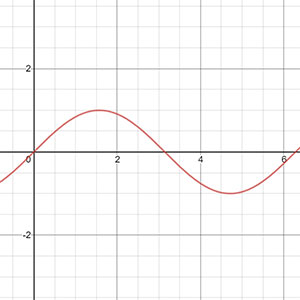y = sin x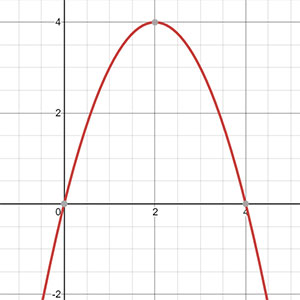y = 4x – x2

## Applying the graph equation

Let’s use the sine curve to create our projectile arc. Add the following to your code in Projectile.cs.

```public class Projectile : MonoBehaviour {
public float speed = 8.5f; // Speed of projectile.
public float radius = 1f; // Collision radius.
float radiusSq; // Radius squared; optimisation.
Transform target; // Who we are homing at.

Vector3 currentPosition; // Store the current position we are at.
float heightOffset; // Projectile height offset.
float distanceTravelled; // Record the distance travelled.

void OnEnable() {
// Pre-compute the value.
radiusSq = radius * radius;
currentPosition = transform.position;
}

void Update() {
// If there is no target, destroy itself and end execution.
if ( !target ) {
Destroy(gameObject);
// Write your own code to spawn an explosion / splat effect.
return; // Stops executing this function.
}

// Move ourselves towards the target at every frame.
Vector3 direction = target.position - transform.position;
//transform.position += direction.normalized * speed * Time.deltaTime;
currentPosition += direction.normalized * speed * Time.deltaTime; // Set where we are at.
distanceTravelled += speed * Time.deltaTime; // Record the distance we are travelling.

// Set our position to <currentPosition>, and add a height offset to it.
float heightOffset = Mathf.Sin(distanceTravelled);
transform.position = currentPosition + new Vector3( 0, 0, heightOffset );

// Destroy the projectile if it is close to the target.
if ( direction.sqrMagnitude < radiusSq ) {
Destroy(gameObject);
// Write your own code to spawn an explosion / splat effect.
// Write your own code to deal damage to the .
}
}

// So that other scripts can use Projectile.Spawn to spawn a projectile.
public static Projectile Spawn(GameObject prefab, Vector3 position, Quaternion rotation, Transform target) {
// Spawn a GameObject based on a prefab, and returns its Projectile component.
GameObject go = Instantiate(prefab, position, rotation);
Projectile p = go.GetComponent<Projectile>();

// Rightfully, we should throw an error here instead of fixing the error for the user.
if(!p) p = go.AddComponent<Projectile>();

// Set the projectile's target, so that it can work.
p.target = target;
return p;
}
}```

This will cause your bullets to move in this fashion, similar to the sine curve, because of the line:

`float heightOffset = Mathf.Sin(distanceTravelled)`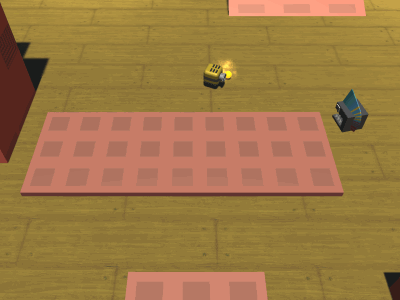Yes, I’ve added a trail to the bullet.

While it looks nice, this is not exactly what we want. We only want this part of the sine curve: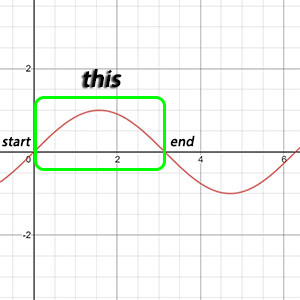Pun intended.

Article continues after the advertisement:

This means that we have to add coefficients to the y = sin x equation, such that the end point of the curve coincides with the location of the target. Here’s an article explaining the sine wave coefficient mumbo-jumbo, if you don’t know what they are.

Mess around with the coefficients (you can use Desmos to do so), and you will find that this is the sine wave equation you want:

y = a sin ( b-1 πx )

where,

x = distance travelled;
y = projectile height;
a = maximum projectile height; and
b = distance between projectile’s spawn location and target

We will need to tie the value of a (maximum projectile height) to the value of b (distance between projectile’s spawn location and target), instead of setting a to a fixed value so that we don’t get unrealistic arcs like these at close range.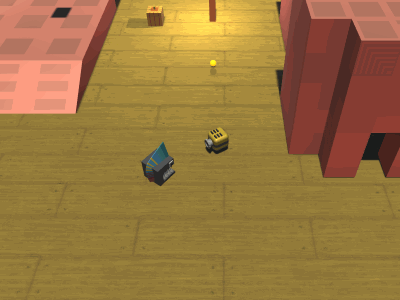We’re not playing Gunbound.

Let a = a1d, where a1 = arc factor,

y = a1b sin ( b-1 πx )

This turns our `heightOffset` line into this:

`float heightOffset = arcFactor * totalDistance * Mathf.Sin( distanceTravelled * Mathf.PI / totalDistance );`

If you’re wondering why b-1 becomes `/ totalDistance` instead of `Mathf.Pow( totalDistance, -1 )`, remember that b-1 = 1 ÷ b. Since divisions compute faster than exponential operations, we choose to divide in our code instead.

Of course, we also have to add a couple more lines to the code to compute all the new variables that have come into play:

```public class Projectile : MonoBehaviour {
public float speed = 8.5f; // Speed of projectile.
public float radius = 1f; // Collision radius.
float radiusSq; // Radius squared; optimisation.
Transform target; // Who we are homing at.

Vector3 currentPosition; // Store the current position we are at.
float distanceTravelled; // Record the distance travelled.

public float arcFactor = 0.5f; // Higher number means bigger arc.
Vector3 origin; // To store where the projectile first spawned.

void OnEnable() {
// Pre-compute the value.
radiusSq = radius * radius;
origin = currentPosition = transform.position;
}

void Update() {
// If there is no target, destroy itself and end execution.
if ( !target ) {
Destroy(gameObject);
// Write your own code to spawn an explosion / splat effect.
return; // Stops executing this function.
}

// Move ourselves towards the target at every frame.
Vector3 direction = target.position - currentPosition;
currentPosition += direction.normalized * speed * Time.deltaTime;
distanceTravelled += speed * Time.deltaTime; // Record the distance we are travelling.

// Set our position to <currentPosition>, and add a height offset to it.
float totalDistance = Vector3.Distance(origin, target.position);
float heightOffset = arcFactor * totalDistance * Mathf.Sin( distanceTravelled * Mathf.PI / totalDistance );
transform.position = currentPosition + new Vector3( 0, 0, heightOffset );

// Destroy the projectile if it is close to the target.
if ( direction.sqrMagnitude < radiusSq ) {
Destroy(gameObject);
// Write your own code to spawn an explosion / splat effect.
// Write your own code to deal damage to the .
}
}

// So that other scripts can use Projectile.Spawn to spawn a projectile.
public static Projectile Spawn(GameObject prefab, Vector3 position, Quaternion rotation, Transform target) {
// Spawn a GameObject based on a prefab, and returns its Projectile component.
GameObject go = Instantiate(prefab, position, rotation);
Projectile p = go.GetComponent<Projectile>();

// Rightfully, we should throw an error here instead of fixing the error for the user.
if(!p) p = go.AddComponent<Projectile>();

// Set the projectile's target, so that it can work.
p.target = target;
return p;
}
}```

This completes our projectile arc!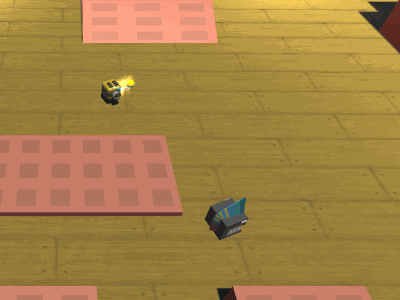Again, nice effects not included.

If you find any issues, or have more effective ways to structure the code, please share them in the comments section below. Should you find parts of the article unclear, please ask about them in the comments section below too!

Article continues after the advertisement: